# \$B%U%!%s!&%M%9WB@1(B

213P/Van Ness (2011)###\$B%W%m%U%#!<%k(B

 \$BId9f(B 213P/2009 B3 \$B8!=PF|(B 2009\$BG/(B1\$B7n(B31\$BF|(B \$B8!=P8wEY(B 20.7\$BEy(B \$B8!=P G. Hug (Scranton, KS, U.S.A.)

###\$B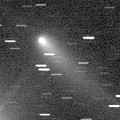###\$B50F;MWAG(B

``` 213P/Van Ness
Epoch 2011 June 8.0 TT = JDT 2455720.5
T 2011 June 16.26367 TT                                 MPC
q   2.1225796            (2000.0)            P               Q
n   0.15574946     Peri.    3.33208     +0.71872845     +0.68289645
a   3.4212516      Node   312.67363     -0.64195641     +0.57956550
e   0.3795898      Incl.   10.23960     -0.26706065     +0.44469795
P   6.33
From 2777 observations 2005 Aug. 4-2012 Jan. 9, mean residual 0".6.

213P-B/Van Ness
Epoch 2011 June 8.0 TT = JDT 2455720.5
T 2011 June 16.41746 TT                                 MPC
q   2.1227068            (2000.0)            P               Q
n   0.15571391     Peri.    3.33752     +0.71874964     +0.68287180
a   3.4217723      Node   312.67004     -0.64194292     +0.57958387
e   0.3796470      Incl.   10.23998     -0.26703604     +0.44471187
P   6.33
From 108 observations 2011 July 12-Sept. 21, mean residual 0".9.

213P-C/Van Ness
Epoch 2011 June 16.0 TT = JDT 2455728.5
T 2011 June 16.37612 TT                                 MPC
q   2.1222713            (2000.0)            P               Q
n   0.15578282     Peri.    3.33122     +0.71860882     +0.68302460
a   3.4207632      Node   312.66460     -0.64206790     +0.57947831
e   0.3795913      Incl.   10.23717     -0.26711456     +0.44461476
P   6.33
From 9 observations 2011 July 29-Sept. 23, mean residual 0".8.

213P-D/Van Ness
T 2011 June 18.29572 TT                                 MPC
q   2.1301594            (2000.0)            P               Q
n   0.15663080     Peri.    4.14276     +0.72857064     +0.67234743
a   3.4084056      Node   312.69499     -0.63345523     +0.58865174
e   0.3750276      Incl.   10.25888     -0.26061331     +0.44881851
P   6.29
From 8 observations 2011 Aug. 19-30.
```

###\$B@1?^(B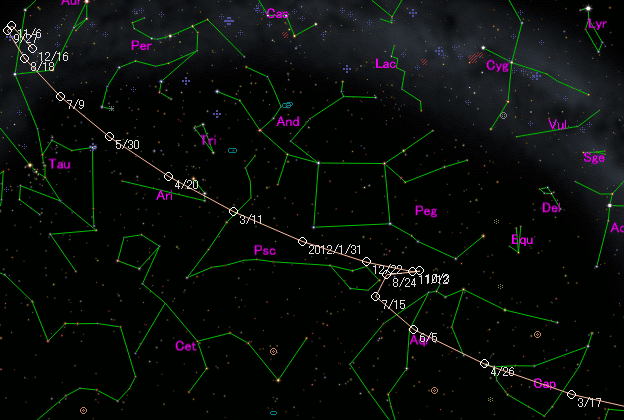###\$B8wEYJQ2=(B

``` 213P/Van Ness
m1 = 12.5 + 5 log\$B&\$(B + 10.0 log r  [    ,-368]  (              \$B!A(B2010\$BG/(B 6\$B7n(B13\$BF|(B)
m1 =  2.5 + 5 log\$B&\$(B + 30   log r  [-368,    ]  (2010\$BG/(B 6\$B7n(B13\$BF|!A(B              )

213P-B/Van Ness
m1 = 15.5 + 5 log\$B&\$(B + 10.0 log r

213P-C/Van Ness
m1 = 17.0 + 5 log\$B&\$(B + 10.0 log r

213P-D/Van Ness
m1 = 17.5 + 5 log\$B&\$(B + 10.0 log r
```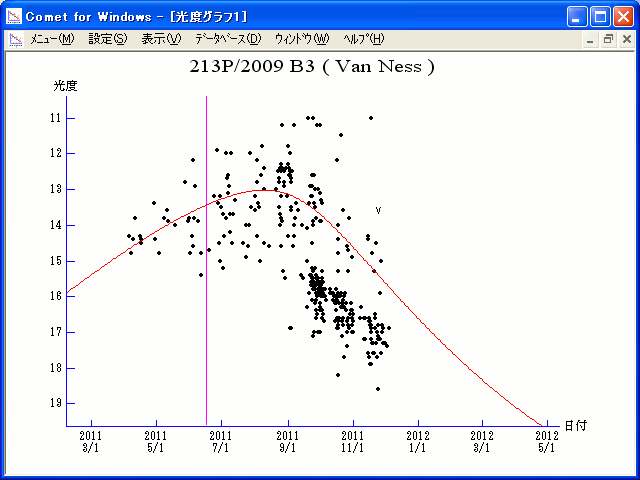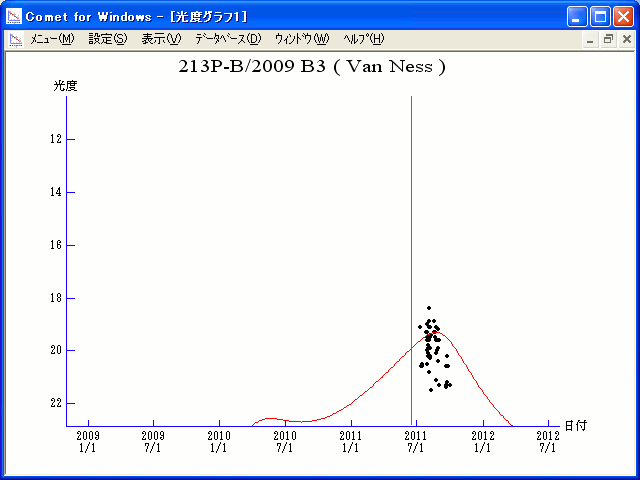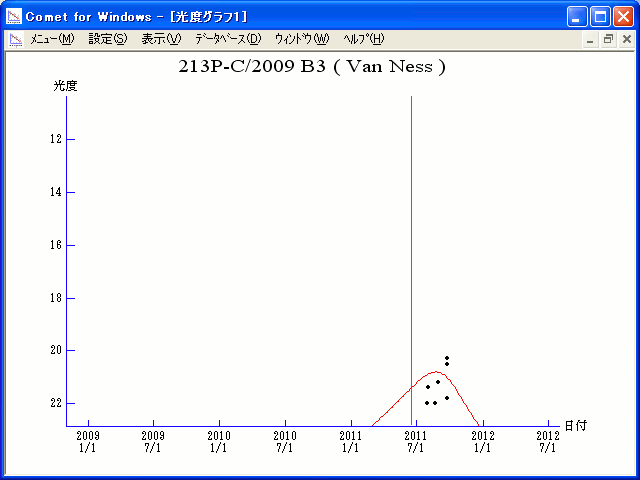##### \$B50F;MWAG\$O(BM.P.E.C. 2012-A52\$B\$K7G:\\$5\$l\$?\$b\$N\$G\$9!#(B B\$B3K\$H(BC\$B3K\$N50F;MWAG\$O(BM.P.E.C. 2011-S56\$B\$K7G:\\$5\$l\$?\$b\$N\$G\$9!#(B D\$B3K\$N50F;MWAG\$O(BM.P.E.C. 2011-S107\$B\$K7G:\\$5\$l\$?\$b\$N\$G\$9!#(B \$B@1?^\$O%9%F%i%J%S%2!<%?(B Ver.8 (\$B%"%9%H%m%"!<%D(B) \$B\$G:n@.\$7\$?\$b\$N\$G\$9!#(B \$B8wEY%0%i%U\$O(BComet for Windows\$B\$G:n@.\$7\$?\$b\$N\$G\$9!#(B